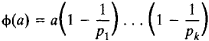# Euler Phi-Function

## Euler Phi-Function

The Euler phi-function of the natural number a is the number φ(a) of natural numbers smaller than a and relatively prime to a:where pl, . . . . . ., pk are the prime factors of a. The function was introduced by L. Euler in 1760 and 1761. If the numbers a and b are relatively prime, then φ(ab) = φ(a)φ(b). Euler’s theorem states that if m > 1 and the greatest common divisor of a and m is 1 (that is, a and m are relatively prime), then the congruence aφ(m) ≡ 1 (mod m) holds. Euler’s phi-function is encountered in many problems of number theory.

Site: Follow: Share:
Open / Close